## Introduction

More and more non-hermitian systems are becoming experimentally accessible1. Therefore, it has become evident that questions concerning foundations of quantum mechanics are no longer only of academic interest. Recent experiments have demonstrated that hermiticity may not be as fundamental as mandated by quantum mechanics2,3. For instance, in4 a spontaneous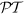-symmetry breaking has been observed indicating a condition weaker than hermiticity (namely5) being realized in nature. Furthermore, in6 exceptional eigenenergies of complex value have been measured challenging the reality of the spectrum imposed by hermiticity.

Conventional quantum mechanics is built upon the Dirac-von Neumann axioms2,3. These state that if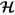is a complex Hilbert space of countable, infinite dimension, then (i) observables of a quantum system are defined as hermitian operators O on, (ii) quantum states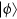are unit vectors inand (iii) the expectation value of an observable O in a stateis given by the inner product, 〈O〉 = 〈ϕ|〉. Interestingly, only axioms (ii) and (iii) are of mathematical necessity needed for a proper probabilistic, physical theory. To demand, however, that any quantum mechanical theory has to be built on hermitian operators is rather mathematically convenient than being fundamentally necessary5,7.

In particular, the restriction to hermitian observables excludes the description of, for instance, quantum field theories with-symmetry, cases where the language of quantum mechanics is used for problems within classical statistical mechanics or diffusion in biological systems, or cases where effective complex potentials are introduced to describe interactions at edges8. Particularly striking examples are optical systems with complex index of refraction. Imagine, for instance, polarized light in a stratified, nontransparent, biaxially anisotropic, dielectric medium warped cyclically along the propagation direction. For such systems it has been shown9 that not only a non-hermitian description becomes necessary, but also that physical intuition has to be invoked carefully. For instance, Berry highlighted9 that adiabatic intuition can be countered dramatically for systems with non-hermitian Hamiltonians.

Very recently, it has become evident that for a special class of non-hermitian systems, namely in-symmetric quantum mechanics10, the quantum Jarzynski equality holds without modification11. For isolated quantum systems evolving under unitary dynamics the so-called two-time energy measurement approach has proven to be practical and powerful. In this paradigm, quantum work is determined by projective energy measurements at the beginning and the end of a process induced by an externally controlled Hamiltonian. The Jarzynski equality12 together with subsequent Nonequilibrium Work Theorems, such as the Crooks fluctuation theorem13, is undoubtedly among the most important breakthroughs in modern Statistical Physics14. Jarzynski showed that for isothermal processes the second law of thermodynamics can be formulated as an equality, no matter how far from equilibrium the system is driven12, 〈exp(−βW)〉 = exp(−βΔF). Here β is the inverse temperature of the environment and ΔF is the free energy difference, i.e., the work performed during an infinitely slow process. The angular brackets denote the average over an ensemble of finite-time realizations of the process characterized by their nonequilibrium work W.

The present study is dedicated to an even more fundamental question. In the following we will analyze to what extent quantum thermodynamic relations are immune to the underlying mathematical formulation of quantum mechanics. Contrary to different studies (see e.g.11) conducted on a similar subject we present the broadest possible class of non-hermitian systems that still allows a thermodynamic theory in the “conventional” sense.

As a main result we will prove that equilibrium as well as non-equilibrium identities of quantum thermodynamics hold, without modification also for quantum systems described by pseudo-hermitian Hamiltonians15. Those systems have either entirely real spectrum or complex eigenvalues appear in complex conjugate pairs. In particular, we will show that the Carnot statement of the second law of thermodynamics holds for any such system and that the quantum Jarzynski equality is not violated as long as the eigenvalue spectrum is real. If the two-time energy measurement could be realized e.g. in a microcavity6, then the Jarzynski equality for pseudo-hermitian systems could be put into a test (see Discussion).

## Fundamentals of Pseudo-Hermitian Quantum Mechanics

To address physical properties of recent experiments4,6 we start by briefly reviewing the mathematical foundations of pseudo-hermitian quantum mechanics8. Let H be a general, non-hermitian Hamiltonian of a physical system and we assume for the sake of simplicity that the spectrum of H, {En}, is discrete (possibly degenerate). Such a Hamiltonian is of physical relevance only if it is measurable, i.e., if a representation of the eigenbasis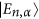is experimentally accessible. Then H is diagonal in this basis. Here n is the quantum number and α counts possible degeneracy. Diagonalizability of H is equivalent to the existence of biorthonormal set of left,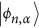and right,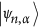, eigenvectors16. In general, the energy eigenvalues are complex and the eigenvalue problem reads15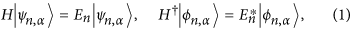with 〈ψnm〉 = δmnδαβ and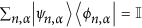. A non-hermitian Hamiltonian such as (1) is called pseudo-hermitian if a g exists such that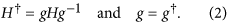It does exist if and only if either all eigenenergies are real or complex ones appear in conjugate pairs with the same degeneracy15. If none of those criteria are met H is generally non-hermitian8; yet it still can be useful, e.g. for an effective description of open quantum systems17. However, when heat is exchanged the two-time energy measurement can no longer describe the work done during a thermodynamic process. Therefore we shall not focus on such cases here. Another interesting class relates to systems that interact with environments, but do not exchange heat. This phenomenon is called dephasing (loss of information)18. For such systems, work can still be determined by the two-time energy measurement and the Jarzynski equality holds as well19,20,21.

Condition (2) assures that H is, in fact, hermitian however with respect to a new inner product, namely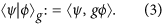Note that g always exists such that 〈ψ|ϕ〉g is positive-definite (this is a genuine inner product) and it can be found if and only if the spectrum of H is real. To make a consistent definition of work for a quantum system within the two–time energy measurement paradigm its spectrum has to be real. Therefore, unless stated otherwise, we shall always assume this to be the case. Then, Eq. (2) can be fulfilled by the following positive-definite operators (g is a proper metric operator)22Often, g fulfilling (2) can be deduced easily from physical properties such as the parity reflection or time reversal23. Nevertheless, only Eq. (4) assures that 〈ψ|ψ〉g > 0 for all states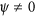. This means that the proper metric may reflect “symmetries” that are hidden from the observer24,25. For instance, if a rotation V exists such that V−1HV is diagonal in an orthonormal basis, then g = VV. This follows directly from Eq. (4). The last formula is especially useful in practice. It allows one to find the metric by analyzing an experimental setup (e.g. inspecting the orientation of the axis, etc.).

In the following we only consider cases where changes of the Hamiltonian are induced by a time–dependent thermodynamic process λt, that is to say Ht = Ht). If such changes occur then the metric operator satisfying Eq. (2) is time-dependent. Nevertheless, the dynamics is still governed by a time-dependent Schrödinger equation. However, a slight modification becomes necessary to preserve unitarity26,27,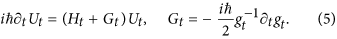Above, ∂t denotes the derivative with respect to time t. The Schrödinger equation (5) can also be rewritten in the standard form, that is, with Ht being the generator. Indeed, it is sufficient to replace ∂t with a covariant derivative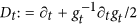28. By construction the unique solution to Eq. (5) obeys the relation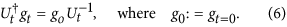This relation can be viewed as the corresponding unitarity condition similar to the “standard” one, i.e.,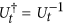.

For pseudo-hermitian systems an average value of a non-hermitian observable A, tr{A}, can be computed as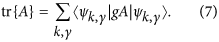Formally, this suggests one to use the following Dirac correspondence between bra and ket vectors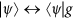16.

### Pseudo–hermitian Jarzynski equality

Having analyzed the mathematical structure of pseudo-hermitian quantum systems, we turn to the physical description to analyze the Jarzynski equality. Without loss of generality and to simplify our notation we assume the spectrum to be non-degenerate.

For an isolated quantum system, the work done during a thermodynamic process λt of duration τ is commonly determined by a two-time energy measurement29. At t = 0 a projective energy measurement is performed. Next, the system evolves unitarily under the generalized time-dependent Schrödinger equation (5) only to be measured again at t = τ. By averaging over an ensemble of realizations of such processes one can reconstruct the distribution of work values30,31,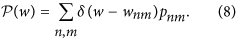Above, pnm denotes a probability that a specific transition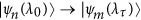will occur, whereas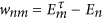is the corresponding work done during this transition. It is important to stress that this work is associated with Ht rather than Ht + Gt as Gt is a gauge field and hence it can have no influence on physical observables32.

The transition probability pnm can be seen as the joint probability that the first measurement will yield the energy value En given the system has been initially prepared in a state ρ0 and the probability that the outcome of the second measurement will be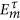given the initial state ψn. Therefore,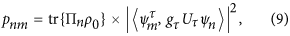where Uτ denotes the evolution operator generated by Ht + Gt at time t = τ, whereas Πn = 〈ψn, g0 〉ψn is the projector into the space spanned by the nth eigenstate. Since Πn is not hermitian the formula for probabilities pnm accounts for the metric g and hence differs from the one usually adopted for hermitian systems31.

Assume the system is initially in a Gibbs state, that is ρ0 = exp(−βH0)/Z0 with Z0 = tr{exp(−βH0)} being the partition function, then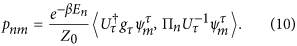To obtain the last expression for pnm we have also invoked the unitarity condition (6). Now, the average exponentiated work can be expressed as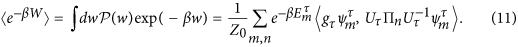Finally, summing out all projectors Πn and taking into account that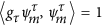we arrive at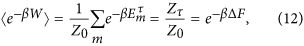where F = (−1/β) ln(Z) is the system’s free energy.

The last equation shows that the Jarzynski equality holds also for non-hermitian systems that admit real spectrum. This is our first main result. Jarzynski has shown that the second law of thermodynamics for isothermal processes can be expressed as an equality arbitrarily far from equilibrium. Our analysis has shown that his result is true for all non-hermitian systems with real spectrum.

### Carnot bound

In the preceding section we argued that if the two-time energy measurement can be performed on a non-hermitian quantum system, then the Jarzynski equality holds as long as the eigenenergies are real. Now, we will prove that the Carnot statement of the second law is also true for all pseudo-hermitian systems.

Consider a generic system that operates between two heat reservoirs with hot, Th and cold, Tc, temperatures, respectively. Then, the Carnot engine consists of two isothermal processes during which the system absorbs or exhausts heat and two thermodynamically adiabatic, that is, isentropic strokes while the extensive control parameter λ is varied33,34. It is well established that the maximum efficiency η for classical systems, attained in the quasistatic limit, is given by the Carnot bound35,36,37: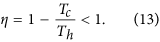Recent years have witnessed an abundance of research38,39,40 investigating whether quantum correlations can be harnessed to break this limit. Recently, the Carnot limit has been proven to be universal within the usual framework33. This limit can be seen as yet another formulation of the second law of thermodynamics for quasistatic processes. We will show that it holds for all pseudo-hermitian systems whether their spectrum is real or not.

We begin by proving that both the energy E = tr{ρH} and entropy S are real in our present framework. Indeed, from (2) it immediately follows that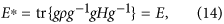with ρ being a Gibbs thermal state. Interestingly, this result holds true even if some of the eigenvalues En are complex. Note, in that case g exists but is not positive definite and thus cannot be expressed like in Eq. (4).

To understand why Eq. (14) holds when complex eigenvalues appear in conjugate pairs note that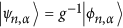and consider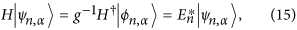showing that if En is in the spectrum of H so is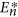. Moreover g−1 maps the subspace spanned by all eigenvectors belonging to En to that belonging to. Since g−1 is invertible, the mapping is one-to-one and the multiplicity of both En andis the same. An interesting realization of such systems is the non-hermitian tight-binding model41.

The result (14) can also be obtained directly, that is, without invoking the metric g explicitly. Indeed, we have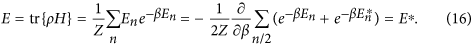In the present case, the thermodynamic entropy is given by the von Neumann entropy42. The latter can be further simplified and it takes the well known form S = β(E − F)33. Since the partition function Z is real so is the free energy F. Hence, we conclude that the entropy S is real.

According to the first law of thermodynamics43, dE = δQ + δW, there are two forms of energy: heat δQ is the change of internal energy associated with a change of entropy, whereas work δW is the change of internal energy due to the change of an extensive parameter, i.e., change of the Hamiltonian of the system. To identify those contributions we write33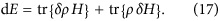In the quasistatic regime, the second law of thermodynamics for isothermal processes states that dS = βδQ. Combining the latter with (17) proves that (i) δQ and thus δW are real and (ii) the intuitive definitions of heat and work introduced in Ref. 44 apply also to pseudo-hermitian systems.

After completing a cycle, a quantum pseudo-hermitian heat engine has performed work 〈W〉 = 〈Qh〉 − 〈Qc〉 and exhausted a portion of heat 〈Qc〉 to the cold reservoir. Therefore, the efficiency of such a device is given by33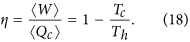In conclusion, we have shown that the Carnot bound, which expresses the second law of thermodynamics for quasistatic processes, holds for all pseudo-hermitian systems. In contrast, the second law for arbitrarily fast processes encoded in the Jarzynski equality (12), only holds for all non-hermitian systems with real spectrum.

## Discussion

### Example 1a

We begin with a model for localization effects in solid state physics41. The general form of its Hamiltonian in one dimension reads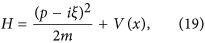where V(x) is a confining potential and p and x are the momentum and position operators respectively. They obey the canonical commutation relation [x, p] = . Real parameter ξ expresses an external magnetic field and m is the mass. Using the Baker-Campbell-Hausdorff formula one can verify that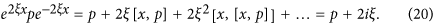Therefore, since [V(x), e2ξx] = 0, we conclude that H is pseudo-hermitian. The metric g = e2ξx is positive definite and thus the spectrum of (19) is real. Further, we assume that the corresponding classical potential Vc(x) has a non-vanishing second derivative and a minimum at x = 0 (e.g.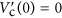). Then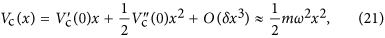where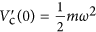has been introduced. After quantization, the eigenvalues and eigenvectors of this non-hermitian harmonic oscillator read (for the sake of simplicity we set m = ħ = 1 throughout)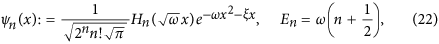where Hn(x) are the Hermite polynomials.

Now we assume that the size of this harmonic trap (e.g. ω) is changed and thus g does not depend on time. Experimentally, harmonic traps are sensitive to initial excitations resulting for a discontinuity of the protocol itself at the beginning45. The most common way to minimize this effect, while quenching between ωi and ωf, is to use functions smooth enough at the “edges”, for instance,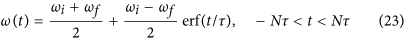where erf() denotes the error function, τ is a time scale and N is an integer emulating infinity. The transition probabilities (9) can be expressed via the following integral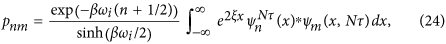where the partition function Z0 = 1/sinh(βωi/2) has been calculated exactly; and ψm(x, ) = Uψn(x) is the solution of Eq. (5), with the initial condition given by (22), at t = . Although ψm(x, ) cannot be obtained analytically, a closed form expressed in terms of a solution to the corresponding classical equation of motion can be found (see e.g. Ref. 46).

Figure 1 (Left panel) shows the average exponentiated work 〈eβW〉 (blue curve) as a function of the number of terms Nmax included in the summation (11). This function quickly converges to e−βΔF proving that the Jarzynski equality (12) holds. On the right panel we have depicted the irreversible work 〈Wirr〉 = 〈W〉 − ΔF (blue curve) as a function of τ which determines the speed at which the energy is supplied to the system. When τ → ∞ the system enters its quasistatic regime and the irreversible work becomes negligible, that is 〈Wirr〉 → 047,48. The inset (red curve) shows the irreversible work calculated for a linear protocol, ω(t) = ωi + (ωf − ωi)t/τ. As we can see, it takes longer for the system to reach its quasistatic regime. Moreover, the oscillatory behavior is a signature of the initial excitation which dominates for fast quenches (small τ).

### Example 1b

Another class of systems that is used to explain localization effects relates to non-hermitian tight-binding models49,50. For example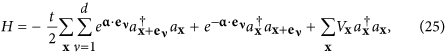where,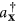and ax are bosonic creation and annihilation operators respectively, eν are the unit lattice vectors and t is the hopping parameter and Vx denotes the on-site potential. Interestingly, the complex eigenvectors appear in conjugate pairs (see Eq. (2) in Ref. 41 and the discussion that follows). Therefore, this model provides another example for a building block of a non-hermitian Carnot engine.

### Example 2

The remainder of the present work is dedicated to a careful study of a second, experimentally relevant example6. Consider a two level system described by the Hamiltonian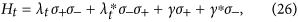where λt is a complex control parameter and γ is a complex constant, whereas σ+ and σ are the raising and lowering fermionic operators. This simple model (26) has been extensively studied in the literature11,51,52 and it has been also realized experimentally both in optics4 and semiconductor microcavities6.

To make the spectrum of (26) real we set λt to be purely imaginary (λt → t); and without any loss of generality we choose γ = 1. This corresponds to the following parameters E1,2 = 0, Γ1,2 = ±λt and q = γ = 1 for the hybrid light–matter system of quasiparticles investigated in Ref. 6. Such systems are formed as a result of a strong interaction between excitons and photons in a semiconductor microcavity53. They are commonly referred to as exciton–polaritons54.

A simple calculation shows that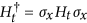, where σx is the Pauli matrix in x direction. Thus Ht is indeed pseudo-hermitian. However, the corresponding σx is not a metric. For instance 〈e1, σxe1〉 = 0, where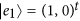. Nevertheless, we can easily find one by rewriting Ht in its diagonal form,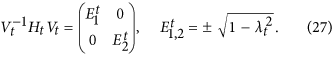Note, both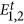are real as long as λt ≤ 1, otherwise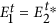. Therefore, the Carnot bound (13) holds in both these regimes, whereas the Jarzynski equality (12) only in the first one. Now, the proper metric can be defined via the similarity transformation Vt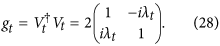To investigate the dynamics of (26) we assume that λt changes on a time scale τ in a linear manner, that is λt = λi + (λf − λi)t/τ. The linearity does not pose any restriction on our analysis as the Jarzynski equality holds for all protocols λt11. Figure 2 (Left panel) depicts the relaxation time Tr = Δ−1, where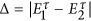, as a function of the final value λf55. The relaxation time diverges as λf approaches the critical point at λ = 1. Similar behavior has been observed for the irreversible work 〈Wirr〉 := 〈W〉 − ΔF in-symmetric systems11. The critical point separates the unbroken domain, where energies are real, from the broken one characterized by complex energy values. The energetic cost associated with a potential crossover between those two regimes becomes infinite and the system “freezes out” before even having a chance to cross to the other regime56,57.